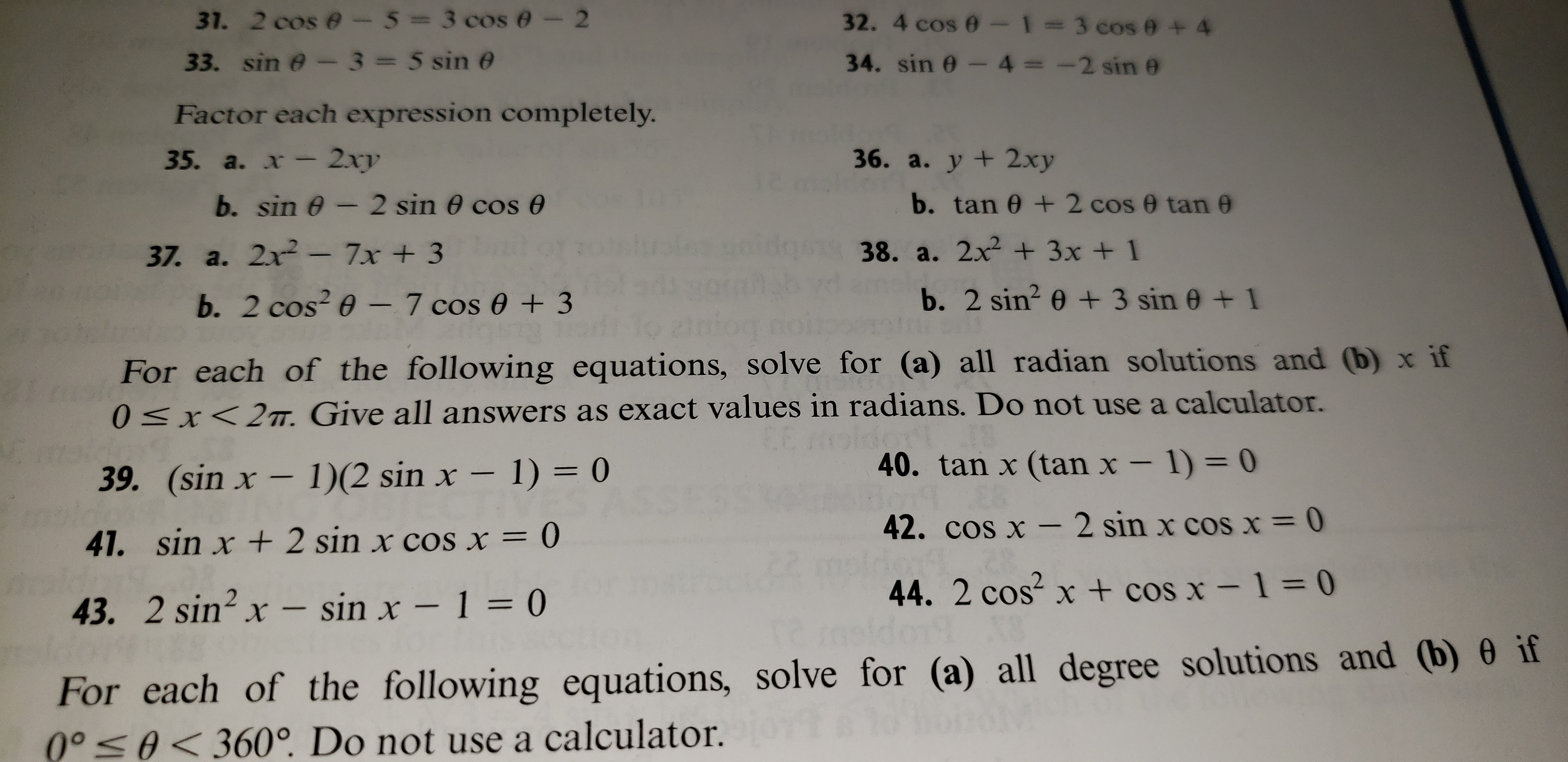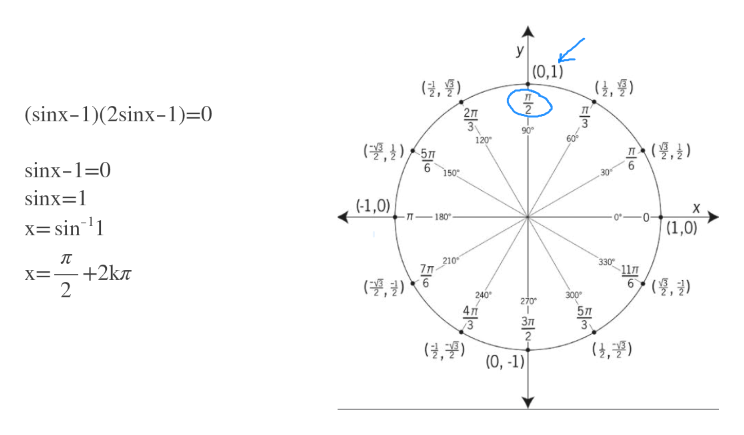# 31. 2 cos e - 5=3 cos e - 233. sin e- 3 5 sin e32. 4 cos e - 1=3 cos e + 434. sin 0 -4 = -2 sin eFactor each expression completely.x- 2xy36. a. у + 2ху35. a.b. sin e- 2 sin 0 cos eb. tan 0 2 cos e tan 038. a. 2x + 3х + 137. a. 2x-7x + 3b. 2 sin2 e+ 3 sin 0+1b. 2 cos 0 -7 cos 0 3For each of the following equations, solve for (a) all radian solutions and (b) x if0 x< 2mT. Give all answers as exact values in radians. Do not use a calculator.40. tan x (tan x - 1) = 039. (sin x - 1) (2 sin x - 1) = 042. cos x -2 sin x cosx = 041. sin x +2 sin x cos x = 044. 2 cos x+ cos x- 1 = 043. 2 sin2 x- sin x - 1 = 0For each of the following equations, solve for (a) all degree solutions and (b) 0 if000

Question
5 views

number 39

(sin x-1)(2sinx-1)=0

what is the turn around time on getting answers?help_outlineImage Transcriptionclose31. 2 cos e - 5=3 cos e - 2 33. sin e- 3 5 sin e 32. 4 cos e - 1=3 cos e + 4 34. sin 0 -4 = -2 sin e Factor each expression completely. x- 2xy 36. a. у + 2ху 35. a. b. sin e- 2 sin 0 cos e b. tan 0 2 cos e tan 0 38. a. 2x + 3х + 1 37. a. 2x-7x + 3 b. 2 sin2 e+ 3 sin 0+1 b. 2 cos 0 -7 cos 0 3 For each of the following equations, solve for (a) all radian solutions and (b) x if 0 x< 2mT. Give all answers as exact values in radians. Do not use a calculator. 40. tan x (tan x - 1) = 0 39. (sin x - 1) (2 sin x - 1) = 0 42. cos x -2 sin x cosx = 0 41. sin x +2 sin x cos x = 0 44. 2 cos x+ cos x- 1 = 0 43. 2 sin2 x- sin x - 1 = 0 For each of the following equations, solve for (a) all degree solutions and (b) 0 if 000<360°. Do not use a calculator. fullscreen
check_circle

Step 1

Using the zero product property make each factor = 0

From sinx=1 we got x=pi/...help_outlineImage TranscriptioncloseУ (0,1) (, 9) (sinx-1)(2sinx-1)=0 п 2л 3 90 60е 120 д (9,2) ( 5 6 150 6 sinx--0 30 sinx=1 (-1,0) -л-180° х3sin 1 |(1,0) л 210 7п 330 11л X3— +2kл 2 6(9,3) 300 240 270 4л 3 Зл 3 (9,) (4,39) (0,-1) fullscreen

### Want to see the full answer?

See Solution

#### Want to see this answer and more?

Solutions are written by subject experts who are available 24/7. Questions are typically answered within 1 hour.*

See Solution
*Response times may vary by subject and question.
Tagged in

### Other